# SSAT Middle Level Math : How to find the area of a square

## Example Questions

← Previous 1

### Example Question #41 : Geometry

What is the area of a square with perimeter 64 inches?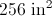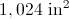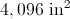It cannot be determined from the information given.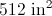Explanation:

The perimeter of a square is four times its sidelength, so a square with perimeter 64 inches has sides with length 16 inches. Use the area formula: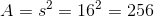### Example Question #42 : Geometry

The area of the square is 81. What is the sum of the lengths of three sides of the square?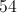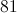Explanation:

A square that has an area of 81 has sides that are the square root of 81 (side2 = area for a square).  Thus each of the four sides is 9.  The sum of three of these sides is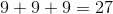.

### Example Question #3 : How To Find The Area Of A Square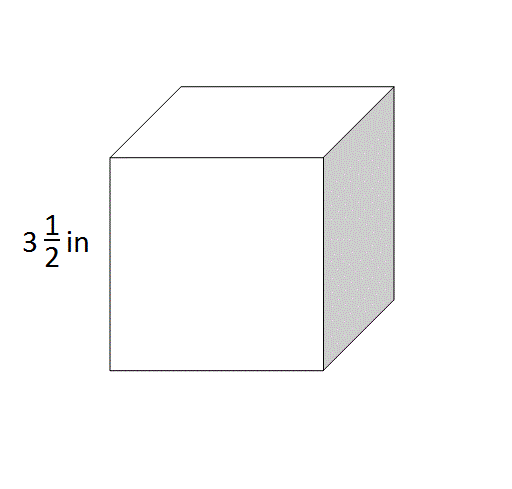What is the total area of the surface of the cube shown in the above diagram?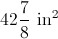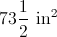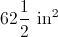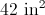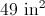Explanation:

A cube comprises six faces, each of which is a square. To find its total surface area, find the area of one face by squaring its sidelength: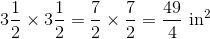Then multiply this by six: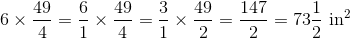### Example Question #1 : How To Find The Area Of A Square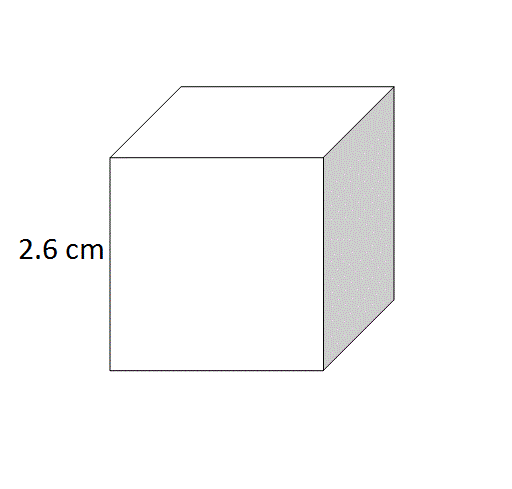What is the total area of the surface of the cube shown in the above diagram?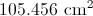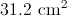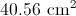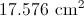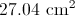Explanation:

A cube comprises six faces, each of which is a square. To find its total surface area, find the area of one face by squaring its sidelength: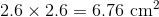Then multiply this by six: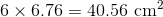### Example Question #5 : How To Find The Area Of A Square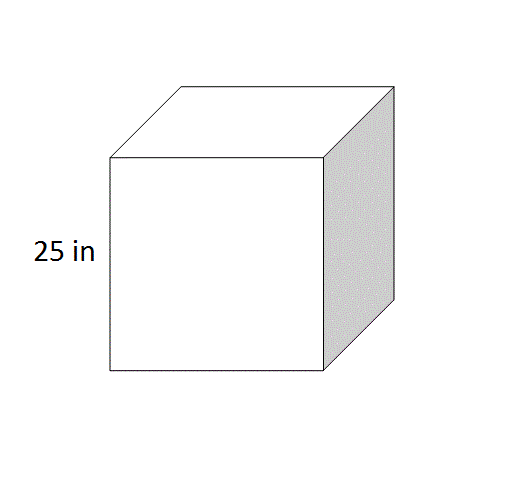What is the total area of the surface of the cube shown in the above diagram?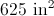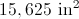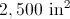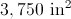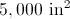Explanation:

A cube comprises six faces, each of which is a square. To find its total surface area, find the area of one face by squaring its sidelength: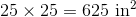Then multiply this by six: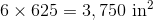### Example Question #1 : How To Find The Area Of A Square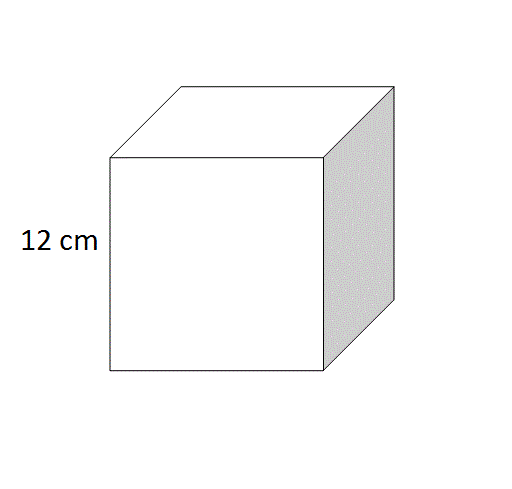What is the total area of the surface of the cube shown in the above diagram?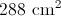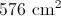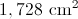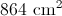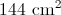Explanation:

A cube comprises six faces, each of which is a square. To find its total surface area, find the area of one face by squaring its sidelength: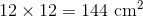Then multiply this by six: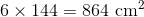### Example Question #4 : How To Find The Area Of A Figure

A square is 9 feet long on each side.  How many smaller squares, each 3 feet on a side can be cut out of the larger square?Explanation:

Each side can be divided into three 3-foot sections.  This gives a total of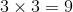squares.  Another way of looking at the problem is that the total area of the large square is 81 and each smaller square has an area of 9.  Dividing 81 by 9 gives the correct answer.

### Example Question #103 : Geometry

Order the following from least area to greatest area:

Figure A: A square with sides of length 3 feet each.

Figure B: A rectangle with length 30 inches and width 42 inches.

Figure C: A rectangle with length 2 feet and width 4 feet.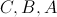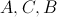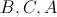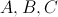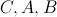Explanation:

Figure A has area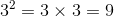square feet.

Figure B has dimensions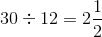feet by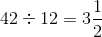feet, so its area is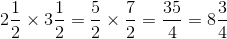square feet.

Figure C has area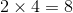square feet.

From least area to greatest, the figures rank C, B, A.

### Example Question #43 : Geometry

The length of one side of a square is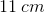. What is the square's area?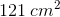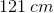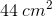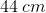Explanation:

The area of any quadrilateral is found by multiplying the length by the width. Because a square has four equal sides, the length and width are the same. For the square in this question, the length and width are.

Remember: area is always given in units2 .

### Example Question #43 : Geometry

If a square has a side that is 3 yards long, what is the area in square feet?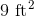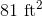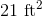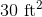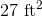Explanation:

The area of a square is found by multiplying the length of a side by itself.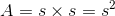If one side is 3 yards, this means one side is 9 feet since there are 3 feet in a yard.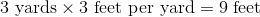Since every side is of equal length, you would multiply 9 feet by 9 feet to find the area.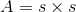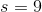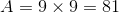This results in 81 square feet, which is the correct answer.

← Previous 1